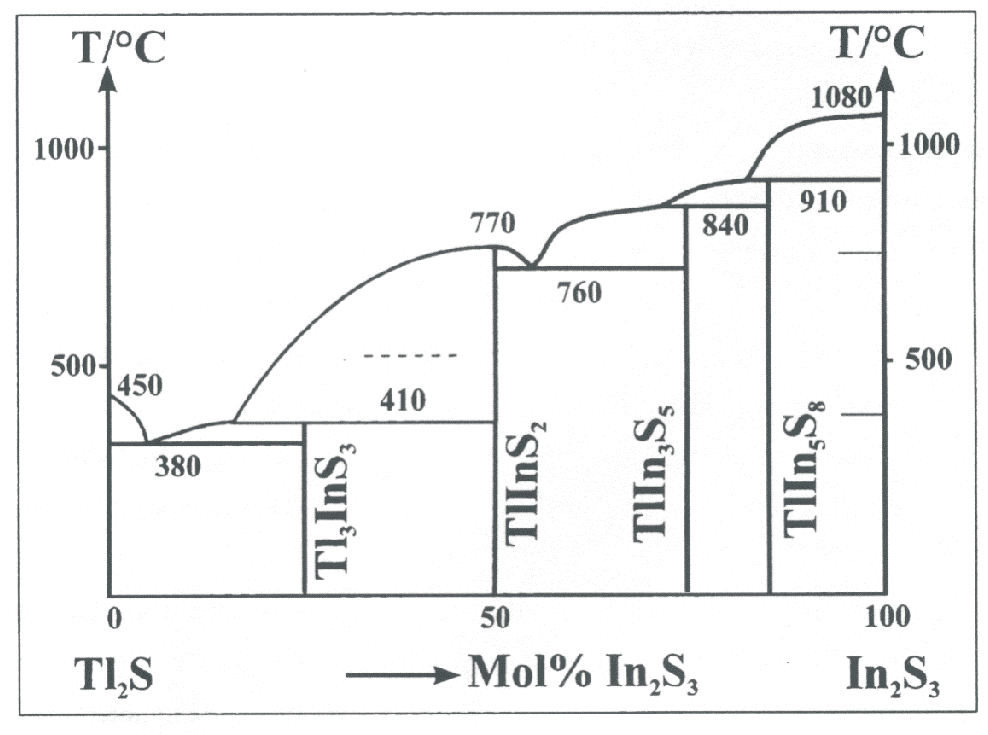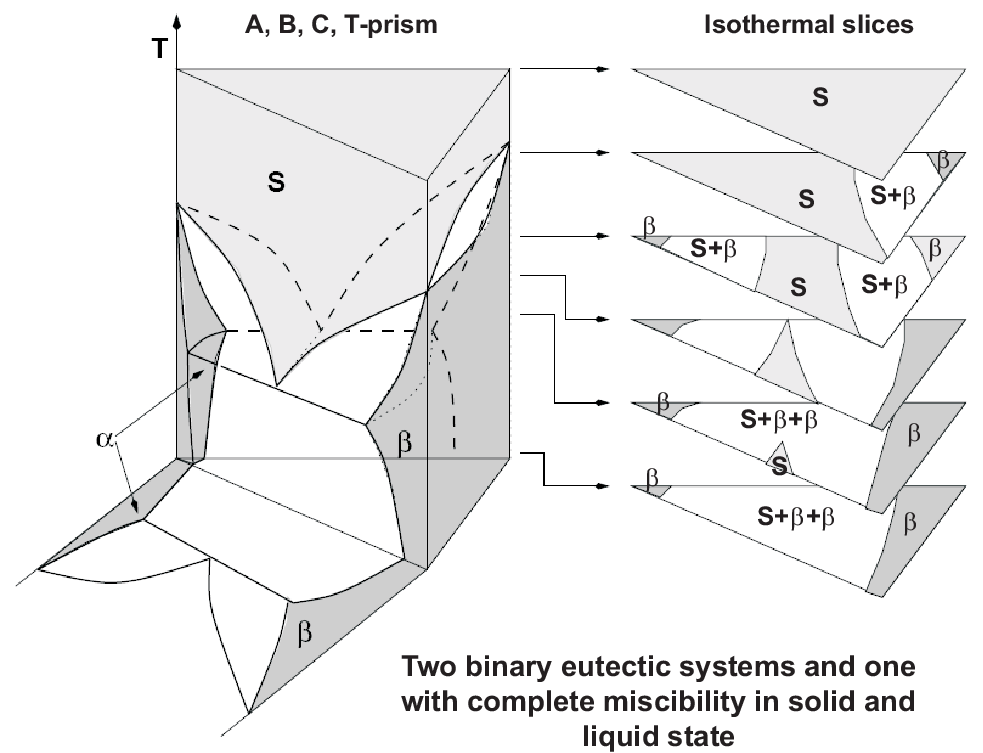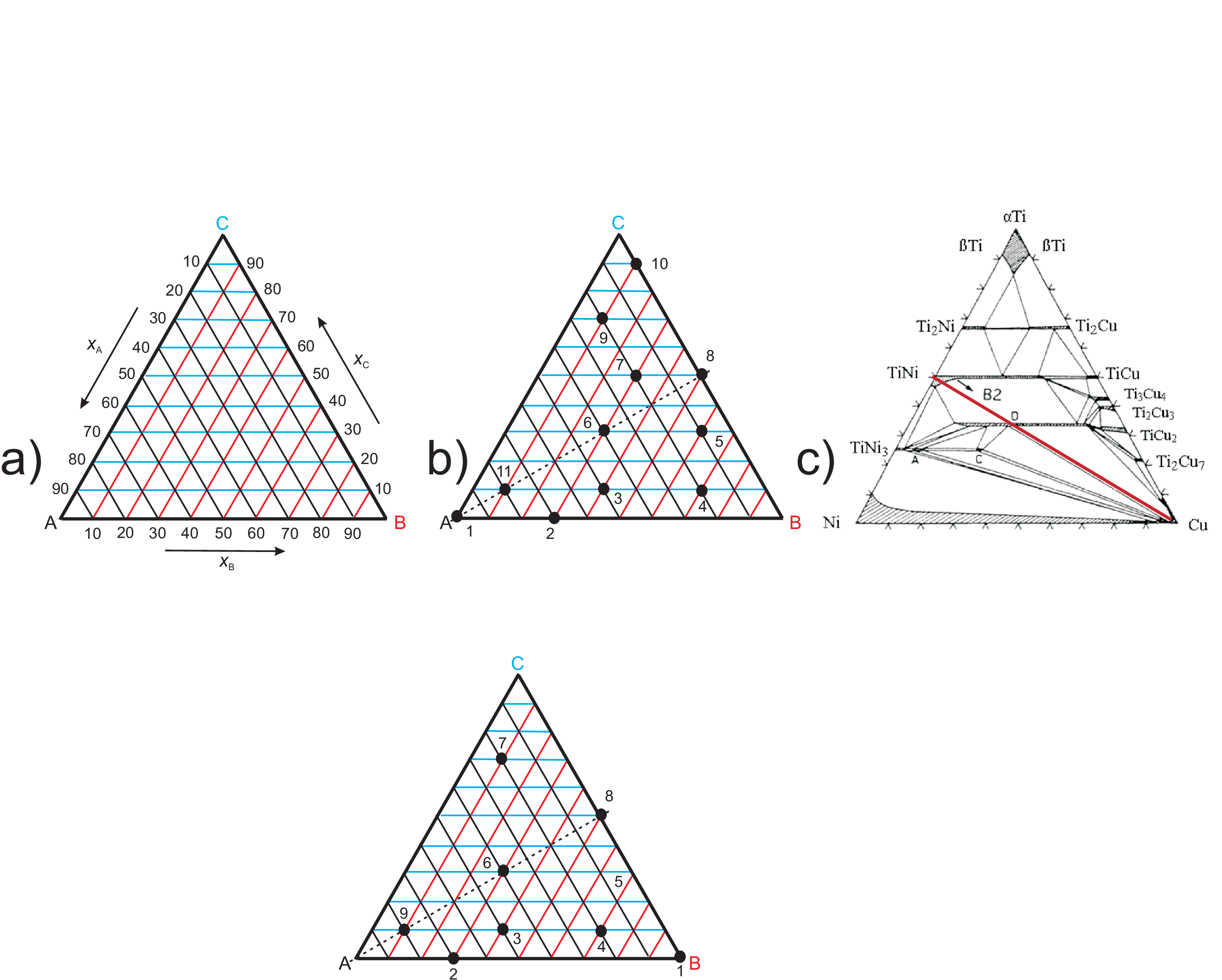### 2.10 Representation of phase diagrams for ternary systems

Since already phase diagrams of binary systems can reveal complicated properties of the mixtures of two components it is quite obvious that a ternary system (mixtures of three constituents) can show much more complicated features of mixing. We will not focus on any detail in what follows but only discuss ways of presenting phase diagram data which at least needs three parameters ($T$ and/or $p$, and the fraction of two components) to specify one point (one thermodynamic state) in a phase diagram.
Fig. 2.14 shows as an example of a pseudo-binary phase diagram a selected path from ”pure” $T{i}_{2}S$ to ”pure” $I{n}_{2}{S}_{3}$, i.e. each ”component” is already a specific composition of two of the three pure components forming the ternary system. Such phase diagrams are highly significant, e.g. for a systematic scan for new ceramics.Figure 2.14: Pseudo-binary phase diagram by taking fractions of specific compositions as $x$-values.Figure 2.15: Schematic representation of isothermal sections in a ternary phase diagram.

In general at least a three dimensional plot is needed to represent phases in a ternary system. A schematic representation of isothermal sections is shown in Fig. 2.15. Most often a set of isothermal slices are chosen, e.g. to illustrate the temperature dependence of different phases.
To characterize the composition of a ternary system the famous Gibbs triangle (as shown in Fig. 2.16) is used which has the following features:

• Any point within the Gibbs triangle represents the overall composition of a ternary system at fixed $T$ and $p$
• Every corner represents a pure condition
• The edges of the triangle represent all possible binary combinations of A, B, and C, thus the fraction of each third component is zero.
• Moving from the opposite edge (0 %) to the corner (100 %), the composition of the component under consideration is increasing gradually and proportionally.
• At the center of the triangle: composition ABC, i.e. 33.33 % of each component
• Sum of molar fractions is one at any pointFigure 2.16: Gibbs triangles. a) Illustrating the percentages of each component; b) Example of several characteristic points; c) Examples of NiTi compositions doped with Cu.

The points marked in Fig. 2.16 b) have molar fractions in percent as shown in the following table:

 1 2 3 4 5 6 7 8 9 10 11 ${x}_{A}$ 100 70 50 20 10 40 20 20 80 ${x}_{B}$ 30 40 70 60 30 30 50 10 10 10 ${x}_{C}$ 10 10 30 30 50 50 70 90 10

Here we will only discuss one example of Cu doping of a NiTi alloy. NiTi is (one of) the most important shape memory alloys, i.e. it can show switching of the shape driven by temperature changes. This needs for a transition from martensitic to austenitic phase and a martensitic micro-structure containing many twin grain boundaries (B2 phase). For binary Ni-Ti the phase field of B2 phase is very narrow and sensitive to slight fluctuations in composition. The compositional field where the B2 phase exists is broader for Cu doped NiTi and thus allows for the production of elastocaloric thin films.

© J. Carstensen (TD Kin II)